An ideal gas is taken from point A to the point B, as shown in the P-V diagram. The work done in the process is -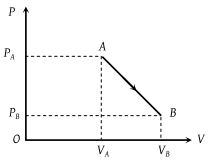(1) $\left({P}_{A}-{P}_{B}\right)\left({V}_{B}-{V}_{A}\right)$

(2) $\frac{1}{2}\left({P}_{B}-{P}_{A}\right)\left({V}_{B}+{V}_{A}\right)$

(3) $\frac{1}{2}\left({P}_{B}-{P}_{A}\right)\left({V}_{B}-{V}_{A}\right)$

(4) $\frac{1}{2}\left({P}_{B}+{P}_{A}\right)\left({V}_{B}-{V}_{A}\right)$

Concept Questions :-

Work done by gas
High Yielding Test Series + Question Bank - NEET 2020

Difficulty Level:

The P-V diagram of a system undergoing thermodynamic transformation is shown in figure. The work done by the system in going from AB C is 30J and 40J heat is given to the system. The change in internal energy between A and C is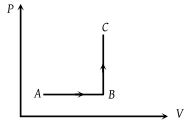(1) 10 J

(2) 70 J

(3) 84 J

(4) 134 J

Concept Questions :-

First law of thermodynamics
High Yielding Test Series + Question Bank - NEET 2020

Difficulty Level:

Consider a process shown in the figure. During this process the work done by the system -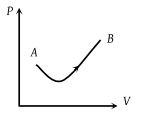(1) Continuously increases

(2) Continuously decreases

(3) First increases, then decreases

(4) First decreases, then increases

Concept Questions :-

Work done by gas
High Yielding Test Series + Question Bank - NEET 2020

Difficulty Level:

Six moles of an ideal gas perform a cycle shown in figure. If the temperature are TA = 600 K, TB = 800 K, TC = 2200 K and TD = 1200 K, the work done per cycle is -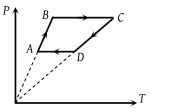(1) 20 kJ

(2) 30 kJ

(3) 40 kJ

(4) 60 kJ

Concept Questions :-

Types of processes
High Yielding Test Series + Question Bank - NEET 2020

Difficulty Level:

Which of the accompanying PV diagrams best represents an isothermal process ?

(1)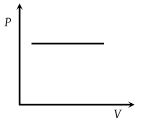(2)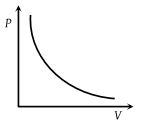(3)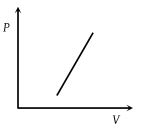(4)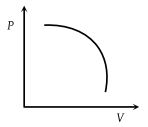Concept Questions :-

Types of processes
High Yielding Test Series + Question Bank - NEET 2020

Difficulty Level:

In the following figure, four curves A, B, C and D are shown. The curves are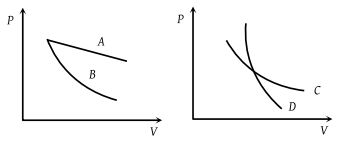(1) Isothermal for A and D while adiabatic for B and C

(2) Adiabatic for A and C while isothermal for B and D

(3) Isothermal for A and B while adiabatic for C and D

(4) Isothermal for A and C while adiabatic for B and D

Concept Questions :-

Types of processes
High Yielding Test Series + Question Bank - NEET 2020

Difficulty Level:

P-V diagram of a cyclic process ABCA is as shown in figure. Choose the correct statement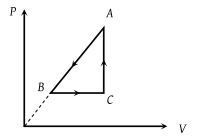(1) $\Delta {Q}_{A\to B}$ = negative

(2) $\Delta {U}_{B\to C}$ = positive

(3) $\Delta {W}_{CAB}$ = negative

(4) All of these

Concept Questions :-

Cyclic process
High Yielding Test Series + Question Bank - NEET 2020

Difficulty Level:

A sample of an ideal gas is taken through a cycle a shown in figure. It absorbs 50J of energy during the process AB, no heat during BC, rejects 70J during CA. 40J of work is done on the gas during BC. Internal energy of gas at A is 1500J, the internal energy at C would be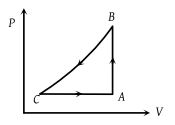(1) 1590 J

(2) 1620 J

(3) 1540 J

(4) 1570 J

Concept Questions :-

Cyclic process
High Yielding Test Series + Question Bank - NEET 2020

Difficulty Level:

In the following P-V diagram two adiabatics cut two isothermals at temperatures T1 and T2 (fig.). The value of $\frac{{V}_{a}}{{V}_{d}}$ will be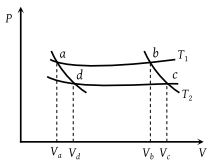(1) $\frac{{V}_{b}}{{V}_{c}}$

(2) $\frac{{V}_{c}}{{V}_{b}}$

(3) $\frac{{V}_{d}}{{V}_{a}}$

(4) VbVc

Concept Questions :-

Types of processes
High Yielding Test Series + Question Bank - NEET 2020

Difficulty Level:

For one complete cycle of a thermodynamic process on a gas as shown in the P-V diagram, which of following is correct ?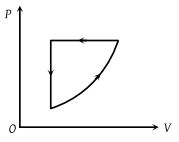(1) $\Delta {E}_{\mathrm{int}}=0,\text{\hspace{0.17em}}Q<0$

(2) $\Delta {E}_{\mathrm{int}}=0,\text{\hspace{0.17em}}Q>0$

(3) $\Delta \text{\hspace{0.17em}}{E}_{\mathrm{int}}>0,\text{\hspace{0.17em}}Q<0$

(4) $\Delta \text{\hspace{0.17em}}{E}_{\mathrm{int}}<0,\text{\hspace{0.17em}}Q>\text{​\hspace{0.17em}}0$

Concept Questions :-

Cyclic process